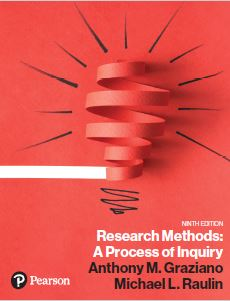﻿ Chapter 5 EssayGraziano & Raulin
Research Methods (9th edition)

## Chapter 5 Essay Questions Statistical Analysis of Data

1. What are the major uses of descriptive and inferential statistics?

2. Explain: "There are several ways of calculating the 'average' of a distribution."

3. What is the major limitation of the range? What makes the variance a better measure?

4. Under what conditions would we use ANOVA?

5. What questions are answered by a correlation coefficient?

6. The concept of variability is extremely important in research. Explain the concept of variability and why it is so important.

7. Which correlation (Spearman r or Pearson r) would be appropriate for each of the following?
(a) relationship of age and rank on an income scale
(b) relationship of height and weight
(c) relationship of finishing position in a race and the length of participants' legs
(e) relationship of body weight and weight of barbell lifted
(f) relationship of ranking in popularity in the sixth grade and ranking in teacher evaluation in the seventh grade

8. What is the concept of negative and positive skewness? Explain, and draw the appropriate graphs.

9. What are the major uses of statistics?

10. What is meant by individual differences, and why is this concept so important in research?

11. What is a standard score, and what information does it provide?

12. What are the populations and samples you would be concerned about in research with adult alcohol abusers?

13. If you were the teaching assistant for this course, how would you explain to the class the concepts of alpha level and Type I and Type II errors?

14. What is meant by the power of a statistical test?

15. With what kind of data do we use frequency distributions?

16. What are the different kinds of summary statistics?

17. Name and define the measures of central tendency and of variability.

18. Is it possible for a distribution of scores to have more than one mode? More than one mean?

19. Why is the mean a better measure of central tendency than the mode?

20. How does a correlation differ from other descriptive statistics?

21. How do inferential statistics differ from descriptive statistics?